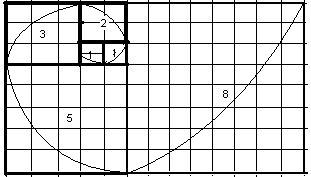# Golden Ratio, Number Calculation

In mathematics, two given quantities are in the golden ratio if their ratio is the same as the ratio of their sum to the larger of the two quantities. The Golden Ratio or golden mean, or golden section describes the perfectly symmetrical relationship between two proportions. It is also known as divine proportion. If the larger quantity divided by smaller quantity is equal to the sum of two quantities divided by the larger quantity than given quantities are called in golden ratio.
In the Fibonacci Sequence (0, 1, 1, 2, 3, 5, 8, 13, ...) each term is the sum of the previous two, and the ratio becomes increasingly closer to the Golden Ratio.
The formula for Golden Ratio = ½(1 + √5) or 1.6180339887499. A/B = (A+B)/A = 1.6180339887499.Enter 1 whole number anyfield A+B : A = : A      : B = :